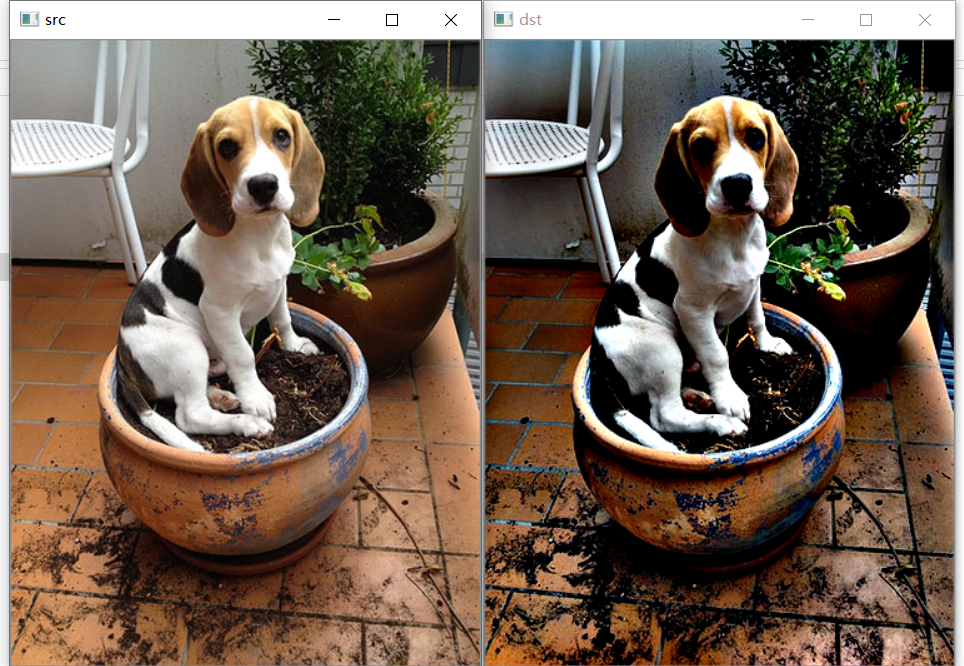2014-12-25 10:27:16 hh555800 阅读数 1074
• ###### OpenCV3.2 Java图像处理视频学习教程

OpenCV3.2 Java图像处理视频培训课程：基于OpenCV新版本3.2.0详细讲述Java OpenCV图像处理部分内容，包括Mat对象使用、图像读写、 基于常用核心API讲述基本原理、使用方法、参数、代码演示、图像处理思路与流程讲授。主要内容包括opencv像素操作、滤波、边缘提取、直线与圆检测、形态学操作与分水岭、图像金子塔融合重建、多尺度模板匹配、opencv人脸检测、OpenCV跟Tomcat使用实现服务器端图像处理服务。

4125 人正在学习 去看看 贾志刚

首先，图像滤波主要涉及线性滤波和非线性滤波两大类。

线性滤波主要有：方框滤波器，均值滤波器，高斯滤波器（注意：滤波器的名字，通常是根据他们的核的使用来命名的，核也可以叫做模板，掩膜，窗口，空间滤波器。名字好多！！！）

非线性滤波器主要有：中值滤波和双边滤波。

1、向量之间的卷积与相关

图像滤波就是让一个图像和一个掩膜去做卷积运算，所以首先我想简单介绍一下一维的卷积运算，以方便的向图像的二维空间进行扩展。

% 实现卷积操作
%   下面分别通过调用matlab代码和自己来实现向量之间的卷积操作
%    涉及到矩阵卷积原理类似，只是实现起来稍微复杂。本程序就不涉及矩阵操作。
% 先实现两个向量的卷积
clc;close all;clear all;
input = [1 1 1 1];  % 供测试代码用
temp = [1 2 3 4];

fprintf('调用库函数imfilter完成相关运算');
imfilter(input, temp, 'conv', 0, 'full')

% 以下自己实现
[m, n] = size(input);
[h ,w] = size(temp);   % 只考虑奇数向量

% 首先将模板旋转180度，注意卷积和相关的区别
temp(1:end) = temp(end : -1: 1);

% 边界通过0的值来扩展
input_temp = zeros(1, n + 2 * ( w - 1 ) );

input_temp(w: w + n - 1) = input(1:end);  % 初始化输入向量

% 让模板滑过扩展的输入向量
kk = size(input_temp, 2) - w  + 1 ;
output = zeros(1, kk);
for i = 1: kk
output(i) = sum ( input_temp(i : i + w - 1) .* temp(1: end) );
end
output

调用库函数imfilter完成相关运算
ans =

1     3     6    10     9     7     4

output =

1     3     6    10     9     7     4


2019-10-18 16:51:30 zbwgycm 阅读数 57
• ###### OpenCV3.2 Java图像处理视频学习教程

OpenCV3.2 Java图像处理视频培训课程：基于OpenCV新版本3.2.0详细讲述Java OpenCV图像处理部分内容，包括Mat对象使用、图像读写、 基于常用核心API讲述基本原理、使用方法、参数、代码演示、图像处理思路与流程讲授。主要内容包括opencv像素操作、滤波、边缘提取、直线与圆检测、形态学操作与分水岭、图像金子塔融合重建、多尺度模板匹配、opencv人脸检测、OpenCV跟Tomcat使用实现服务器端图像处理服务。

4125 人正在学习 去看看 贾志刚

# 基于局部信息的滤波方式

$q_i=a_kI_i+b_k, \forall i \in \omega_k$

$E(a_k,b_k)=\sum_{i\in \omega_k} (a_kI_i+b_k-q_i)^2+\epsilon a^2_k$

# 基于全局优化的滤波方式

$E(x) = f(x,y)+p(x)$

# 总结

2017-03-09 13:24:23 zhangyibo123456789 阅读数 9526
• ###### OpenCV3.2 Java图像处理视频学习教程

OpenCV3.2 Java图像处理视频培训课程：基于OpenCV新版本3.2.0详细讲述Java OpenCV图像处理部分内容，包括Mat对象使用、图像读写、 基于常用核心API讲述基本原理、使用方法、参数、代码演示、图像处理思路与流程讲授。主要内容包括opencv像素操作、滤波、边缘提取、直线与圆检测、形态学操作与分水岭、图像金子塔融合重建、多尺度模板匹配、opencv人脸检测、OpenCV跟Tomcat使用实现服务器端图像处理服务。

4125 人正在学习 去看看 贾志刚

# 浅析matlab图像滤波

1、首先我们要知道为什么要给图像滤波？

在尽量保留图像细节特征的条件下对目标图像的噪声进行抑制，是图像预处理中不可缺少的操作，其处理效果的好坏将直接影响到后续图像处理和分析的有效性和可靠性

2、什么是图像滤波？

由于成像系统、传输介质和记录设备等的不完善，数字图像在其形成、传输记录过程中往往会受到多种噪声的污染。另外，在图像处理的某些环节当输入的像对象并不如预想时也会在结果图像中引入噪声。这些噪声在图像上常表现为一引起较强视觉效果的孤立像素点或像素块。一般，噪声信号与要研究的对象不相关它以无用的信息形式出现，扰乱图像的可观测信息。
对于数字图像信号，噪声表为或大或小的极值，这些极值通过加减作用于图像像素的真实灰度值上，对图像造成亮、暗点干扰，极大降低了图像质量，影响图像复原、分割、特征提取、图像识别等后继工作的进行。
要构造一种有效抑制噪声的滤波器必须考虑两个基本问题：能有效地去除目标和背景中的噪声;同时，能很好地保护图像目标的形状、大小及特定的几何和拓扑结构特征

3、常见的滤波器有哪些？

也称均值滤波算法，主要思想为邻域平均法，即用几个像素灰度的平均值来代替每个像素的灰度。有效抑制加性噪声，但容易引起图像模糊，可以对其进行改进，主要避开对景物边缘的平滑处理。

中值滤波由Turky在1971年提出，最初用于时间序列分析，后来被用于图像处理，并在去噪复原中取得了较好的效果。中值滤波器是基于次序统计完成信号恢复的一种典型的非线性滤波器，其基本原理是把图像或序列中心点位置的值用该域的中值替代，具有运算简单、速度快、除噪效果好等优点，曾被认为是非线性滤波的代表。然而，一方面中值滤波因不具有平均作用，在滤除诸如高斯噪声时会严重损失信号的高频信息，使图像的边缘等细节模糊；另一方面中值滤波的滤波效果常受到噪声强度以及滤波窗口的大小和形状等因素的制约，为了使中值滤波器具有更好的细节保护特性及适应性，人们提出了许多中值滤波器的改进算法。
标准中值滤波算法的基本思想是将滤波窗口内的最大值和最小值均视为噪声，用滤波窗口内的中值代替窗口中心像素点的灰度，在一定程度上抑制了噪声。实际上在一定邻域范围内具有最大或最小灰度值这一特性的，除了噪声点，还包括图像中的边缘点、线性特征点等。中值滤波以此作为图像滤波依据，其滤波结果不可避免地会破坏图像的线段、锐角等信息。因此，要找到一种既能实现有效滤除噪声，又能完整保留图像细节的滤波机制，仅考虑噪声的灰度特性是难以实现的。

Wiener维纳滤波：使原始图像和其恢复图像之间的均方误差最小的复原方法，是一种自适应滤波器，根据局部方差来调整滤波器效果。对于去除高斯噪声效果明显。

随着数学各分支在理论和应用上的逐步深入，以数学形态学为代表的非线性滤波在保护图像边缘和细节方面取得了显著进展。形态学滤波器是近年来出现的一类重要的非线性滤波器，它由早期的二值形滤波器发展为后来的多值(灰度)形态滤波器，在形状识别、边缘检测、纹理分析、图像恢复和增强等领域了广泛的应用。
形态滤波方法充分利用形态学运算所具有的几何特征和良好的代数性质，主要采用态学开、闭运算进行滤波操作。从形态学基本原理可知，形态学的开运算会去掉图像上与结构元素的形态不相吻合的相对亮的分布结构，同时保留那些相吻合的部分;而闭运算则会填充那些图像上与结构元素不相吻合的相对暗的分布结构，同时保留那些相吻合的部分。因此他们都可以用来有效的提取特征和平滑像。值得注意地是，采用形态滤波器时，应根据不同的目的选择具有不同形状、大小和方向特性的结构元素。
此外，形态学开、闭运算都具有幂等性，这意味着一次滤波就己将所有特定于结构元素的噪声滤除千净，再次重复不会产生新的结果。这是一个经典方法(如线性卷积滤波、中值滤波)所不具备的性质。由于形态学运算是从图像的几何形态观点来进行图像处理的，因此这种优良的非线性滤波器能在滤波的同时，保持图像结构不被钝化。

4、图像去噪典的型算法及matlab实现

（1）、均值滤波matlab程序参考：
I=imread('C:\Documents and Settings\Administrator\桌面\1.gif');%读取图像

J=imnoise(I,'gaussian',0,0.005);%加入均值为0，方差为0.005的高斯噪声

subplot(2,3,1);imshow(I);

title('原始图像');

subplot(2,3,2); imshow(J);

title('加入高斯噪声之后的图像');

%采用MATLAB中的函数filter2对受噪声干扰的图像进行均值滤波

K1=filter2(fspecial('average',3),J)/255; %模板尺寸为3

K2=filter2(fspecial('average',5),J)/255;% 模板尺寸为5

K3=filter2(fspecial('average',7),J)/255; %模板尺寸为7

K4= filter2(fspecial('average',9),J)/255; %模板尺寸为9

subplot(2,3,3);imshow(K1);

title('改进后的图像1');

subplot(2,3,4); imshow(K2);

title('改进后的图像2');

subplot(2,3,5);imshow(K3);

title('改进后的图像3');

subplot(2,3,6);imshow(K4);

title('改进后的图像4');
（2）、二维自适应维纳滤波matlab程序参考：
I=imread('C:\Documents and Settings\Administrator\桌面\1.gif'); %读取图像

J=imnoise(I,'gaussian',0,0.005); %加入均值为0，方差为0.005的高斯噪声

K2=wiener2(J,[3 3]); %对加噪图像进行二维自适应维纳滤波

K2=wiener2(J,[5 5]); %对加噪图像进行二维自适应维纳滤波

K2=wiener2(J,[7 7]); %对加噪图像进行二维自适应维纳滤波

K2=wiener2(J,[9 9]); %对加噪图像进行二维自适应维纳滤波

subplot(2,3,1);imshow(I);

title('原始图像');

subplot(2,3,2);imshow(J);

title('加噪图像');

subplot(2,3,3);imshow(K1);

title('恢复图像1');

subplot(2,3,4);imshow(K2);

title('恢复图像2');

subplot(2,3,5);imshow(K3);

title('恢复图像3');

subplot(2,3,6);imshow(K4);

title('恢复图像3');

（3）、matlab图像处理形态学滤波教程详细参考：
http://blog.csdn.net/zhangyibo123456789/article/details/60955182

：均值滤波、中值滤波以及二维自适应维纳滤波的详细比较（带图）
http://www.cnblogs.com/xiangshancuizhu/archive/2011/01/04/1925276.html

2019-08-17 22:40:39 csuwoshikunge 阅读数 267
• ###### OpenCV3.2 Java图像处理视频学习教程

OpenCV3.2 Java图像处理视频培训课程：基于OpenCV新版本3.2.0详细讲述Java OpenCV图像处理部分内容，包括Mat对象使用、图像读写、 基于常用核心API讲述基本原理、使用方法、参数、代码演示、图像处理思路与流程讲授。主要内容包括opencv像素操作、滤波、边缘提取、直线与圆检测、形态学操作与分水岭、图像金子塔融合重建、多尺度模板匹配、opencv人脸检测、OpenCV跟Tomcat使用实现服务器端图像处理服务。

4125 人正在学习 去看看 贾志刚

## 图像滤波问题描述

为了内容的完整性，首先对图像滤波进行简单的回顾。令$I\in R^{h\times w\times c}$表示图像，是一个二维矩阵，$h,w$分别表示图像的高度和宽度，$c=1,3$分别表示灰度图像和彩色图像，$I$中的每个元素$I(i,j)$称作一个像素。不失一般性，我们以$c=1$即灰度图为例。本质上图像$I$是一个定义二维网格点上的函数，也即$I:(i,j)\in R^{2} \rightarrow I(i,j)\in R$
$f:I\in R^{h\times w}\rightarrow \hat{I}\in R^{h\times w}$表示滤波器。常见的滤波过程就是：计算每个像素$I(i,j)$经过滤波后的值$\hat{I}(i,j)$，都是通过加权像素$I(i,j)$的邻域像素值，如下公式所示：
$\hat{I}(i,j)=f\circ I(i,j)=\frac{1}{\sum w(i',j')}\sum_{(i',j')\in N} w(i',j')I(i',j')\tag{1}$
其中$N$表示像素$I(i,j)$的邻域（也称滤波器的窗口），注意这里的邻域关系仅仅是像素点在定义域的相邻关系，如图像上的8邻域等。

## 1. 空间(即图像定义域)相邻关系的滤波器

常见的滤波器，如高斯滤波器，均值滤波器等，都属于这一类滤波器。
如(1)式所示，当权重
$w(i',j')=\frac{1}{n}$其中$n=|N|$表示邻域像素的个数， 这就均值滤波器。
当权重
$w(i',j')=\frac{1}{2\pi\sigma^2}e^{-{\frac{(i'-i)^2+(j'-j)^2}{2\sigma^2}}}$
就是通常的高斯滤波器。

注意这类滤波器，在进行图像滤波的时候仅仅考虑图像的定义域上的邻域关系(也即空间上的邻域关系)。这样通常会导致图像模糊，因为仅仅按图像定义域或空间上的相邻关系，会导致空间(或定义域)上相邻但是像素值相差巨大的像素参与滤波过程，如下图所示：按8邻域关系，计算点$A$的高斯滤波或均值滤波时，空间相邻的像素点$B,C,D,E$（注意它们的像素值（白）与$A$点的像素值（黑）相差很大），会参与滤波公式(1)中的加权平均。很显然，滤波后会导致，在$A$点附近的图像边缘会出现模糊。下面就介绍双边滤波，能够在很大程度上避免这一种仅仅依靠图像定义域相邻引起的图像模糊。

## 2. 双边滤波器（bilateral filter）

如何将上图中的点$A$$B,C,D,E$区分开来，使得(1)式中计算$A$点的加权平均时，$B,C,D,E$贡献的权重为0（当然这是理想的情况），就成了关键。那么一个很自然的想法是，同时考虑像素点的像素值，这样将像素点投到高维空间，那么有可能$B,C,D,E$将不再是$A$的相邻点。因为在低维空间中不易区分的点，在高维空间中可能比较容易区分开来。这点类似SVM（支持向量机）中的核函数设置的思想。

同时统计像素点$A$的坐标和像素值，这其实就是图像函数$I:(i,j)\rightarrow I(i,j)\in R$的graph, 记作
$G=\{(i,j,I(i,j)):(i,j)是图像像素坐标\}\tag{2}$
它是一个二维流形（嵌入在三位欧式空间$R^3$中），其实就是一个曲面，显然在这个graph $G$上，$B,C,D,E$$A$不再是相邻点。但是在直接二维流形$G$上寻找点的相邻点可能非常复杂，因为曲面的形状可能很不规则（曲率不为0），不像二维平面网格点$(i,j)$那样容易寻找相邻点。

为了计算方便，退而求其次，把二维曲面$G$嵌入在三维空间中考虑，利用三维欧式空间$R^3$的曲率为0，在三维网格点中寻找相邻点，这样就和在二维网格中种寻找相邻点一样方便。但是考虑到嵌入的$G$实则是函数$I$的图像graph，本质上是二维的，所以双边滤波仍按定义域寻找相邻点即可，但是要减弱这些相邻点中颜色差异较大的点对滤波贡献的权重。

可以看到，在把$G$嵌入三维空间考虑时，$A$点在对应$(i_A,j_A,I_A)$。以$D$点为例，$D$对应$(i_D,j_D,I_D)$。不妨以欧式范数为例，在三维空间中$A,D$之间的距离为
$d(A,D)=\sqrt{(i_D-i_A)^2+(j_D-j_A)^2+(I_D-I_A)^2}=\sqrt{1+1+(I_D-I_A)^2}\tag{3}$
则如上面(3)式所示，那么当$A,D$的像素值之差$|I_D-I_A|$很大时，那么在$G$$d(A,D)$距离就很远。
而双边滤波正是通过，考虑这种距离来弱化$D$$A$的影响，这点可以从下面双边滤波的公式中，得到体现
$\hat{I}_A=\frac{1}{\sum w(q,A)}\sum_{q\in N}w(q,A)I_q\tag{4}$
其中，$N$是点$A$的邻域，
$w(q,A)=e^{-\frac{(i_q-i_A)^2+(j_q-j_A)^2}{2\sigma_{s}^{2}}-\frac{(I_q-I_A)^2}{2\sigma_{r}^2}}$

显然，当$q,A$之间的像素值相差很大时，$w(q,A)$就会变得很小，从而达到了弱化$q$点对$A$点的滤波值的影响，易见上图中的$B,C,D,E$$A$的距离很大（在$R^3$中考虑它们之间的距离时）。所以滤波时，$B,C,D,E$$A$的影响就大大减弱，从而大大减轻滤波后边界模糊的现象，达到了保边滤波的效果。

## 3. 如何快速实现双边滤波

即使双边滤波有很好的保边效果，但是当图像的尺寸以及滤波器的窗口尺寸很大时，双边滤波的速度就可能很慢。双边滤波这不像通常的高斯滤波和均值率波等，双边滤波(4)式中的权重系数$w$是与图像的像素值有关的，因而不能像高斯滤波或者均值滤波那样有固定的模板通过卷积快速实现。后面系列，会介绍快速实现近似双边滤波的相关文章以及在双边滤波在深度学习中的应用。。。。。

: Fast high-dimensional filtering using the permutohedral lattice.

图像处理 图像滤波 相关内容

2019-07-07 22:29:41 wujuxKkoolerter 阅读数 563
• ###### OpenCV3.2 Java图像处理视频学习教程

OpenCV3.2 Java图像处理视频培训课程：基于OpenCV新版本3.2.0详细讲述Java OpenCV图像处理部分内容，包括Mat对象使用、图像读写、 基于常用核心API讲述基本原理、使用方法、参数、代码演示、图像处理思路与流程讲授。主要内容包括opencv像素操作、滤波、边缘提取、直线与圆检测、形态学操作与分水岭、图像金子塔融合重建、多尺度模板匹配、opencv人脸检测、OpenCV跟Tomcat使用实现服务器端图像处理服务。

4125 人正在学习 去看看 贾志刚

# 高斯同态滤波图像增强

同态滤波是一种在频域中同时将图像亮度范围进行压缩并将图像对比度增强的方法。同态滤波增强属于图像频域处理范畴，作用是对图像灰度范围进行调整，通过消除图像上照明不均匀的问题，增强图像的细节，同时对不损失亮区的图像细节。

一幅图像 能够使用它的入射光分量和反射光分量来表示 ，关系如下：
$f(x,y) = i(x,y) \cdot r(x,y)$
另外，入射光分量$i(x,y)$由照明决定，而反射光分量$r(x,y)$则是由物体本身特性决定；入射光分量同傅立叶平面的低频分量相关，而反射光分量则同高频分量相关。
对于$f(x,y) = i(x,y) \cdot r(x,y)$取对数，然后再取傅立叶变换得：
$Z(u,v) = I(u,v) + R(u,v)$
其中，$I(u,v)$$R(u,v)$分别是$\ln[i(x,y)]$$\ln[r(x,y)]$的傅立叶变换，如果选取一个滤波函数$H(u,v)$来处理$Z(u,v)$，则有：
$S(u,v) = Z(u,v)H(u,v) = I(u,v)H(u,v) + R(u,v) * H(u,v)$
其中，$S(u,v)$是滤波后的傅立叶变换。再对取指数即得到最终处理结果$f'(x,y) = \exp(S(u,v))$相当于高通滤波。

处理过程如下：
[外链图片转存失败(img-VrVGc9Ay-1562509670331)(images/03-1.png)]

Python实现代码如下：

def homomorphic_filtering(src,d0 = 10,r1 = 0.5,rh=2,c=4):
gray = src.copy()
if len(src.shape) > 2:
gray = cv2.cvtColor(src,cv2.COLOR_BGR2GRAY)
gray = np.float64(gray)
rows,cols = gray.shape

gray_fft = np.fft.fft2(gray)
gray_fftshift = np.fft.fftshift(gray_fft)
dst_fftshift = np.zeros_like(gray_fftshift)
M,N = np.meshgrid(np.arange(-cols // 2,cols // 2),np.arange(-rows//2,rows//2))
D = np.sqrt(M ** 2 + N ** 2)
Z = (rh - r1) * (1 - np.exp(-c * (D ** 2 / d0 ** 2))) + r1
dst_fftshift = Z * gray_fftshift
dst_ifftshift = np.fft.ifftshift(dst_fftshift)
dst_ifft = np.fft.ifft2(dst_ifftshift)
dst = np.real(dst_ifft)
dst = np.uint8(np.clip(dst,0,255))
return dst



程序运行结果如下：左边为原图像，右边为高斯同态滤波后的结果

图像处理 图像滤波 相关内容

阅读数 133

博文 来自： m0_37772174

阅读数 4323

博文 来自： qq_36511401

阅读数 2334

博文 来自： tongfy0000

阅读数 153

博文 来自： weixin_44225182

阅读数 192

博文 来自： qq_34917728
没有更多推荐了，返回首页